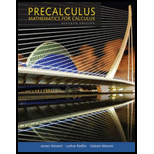Chapter 3.4, Problem 26E### Precalculus: Mathematics for Calcu...

7th Edition
James Stewart + 2 others
ISBN: 9781305071759

#### Solutions

Chapter
Section### Precalculus: Mathematics for Calcu...

7th Edition
James Stewart + 2 others
ISBN: 9781305071759
Textbook Problem

# Integer Zeros All the real zeros of the given polynomial are integers. Find the zeros, and write the polynomial in factored form.26. P(x) = x4 − 2x3 − 3x2 + 8x − 4

To determine

All the real zeroes of a given polynomial and express polynomial in factored form.

Explanation

Given:

The polynomial is P(x)=x42x33x2+8x4 .

Calculation:

The leading coefficient is 1 and constant coefficient is 4 , so the possible real zeros of the

polynomial are factors of 4 : ±1 , ±2 , ±4 .

Test each of the possibilities then substitute the values of x in polynomial P(x) .

To test whether 1 is a zero.

P(x)=x42x33x2+8x4

Substitute 1 for x ,

P(1)=(1)42(1)33(1)2+8(1)4=1+2384=12

Since remainder is 12 , 1 is not a zero of polynomial P(x)=x42x33x2+8x4 .

To test whether 1 is a zero.

P(x)=x42x33x2+8x4

Substitute 1 for x ,

P(1)=(1)42(1)33(1)2+8(1)4=123+84=0

Since remainder is 0 , 1 is a zero of polynomial P(x)=x42x33x2+8x4 .

To test whether 2 is a zero.

P(x)=x42x33x2+8x4

Substitute 2 for x ,

P(2)=(2)42(2)33(2)2+8(2)4=16+1612164=0

Since remainder is 0 , 2 is a zero of polynomial P(x)=x42x33x2+8x4 .

To test whether 2 is a zero.

P(x)=x42x33x2+8x4

Substitute 2 for x ,

P(2)=(2)42(2)33(2)2+8(2)4=161612+164=0

Since remainder is 0 , 2 is a zero of polynomial P(x)=x42x33x2+8x4 .

To test whether 4 is a zero.

P(x)=x42x33x2+8x4

Substitute 4 for x ,

P(4)=(4)42(4)33(4)2+8(4)4=256+12848324=300

Since remainder is 300 , 4 is not a zero of polynomial P(x)=x42x33x2+8x4

### Still sussing out bartleby?

Check out a sample textbook solution.

See a sample solution

#### The Solution to Your Study Problems

Bartleby provides explanations to thousands of textbook problems written by our experts, many with advanced degrees!

Get Started

## Additional Math Solutions

#### In Exercises 13-20, sketch a set of coordinate axes and plot each point. 13. (2, 5)

Applied Calculus for the Managerial, Life, and Social Sciences: A Brief Approach

#### Multiply: 8374203_

Elementary Technical Mathematics

#### For f (x) = sinh x, f (x) = a) cosh2 x b) sinh2 x c) tanh x d) sinh x

Study Guide for Stewart's Single Variable Calculus: Early Transcendentals, 8th